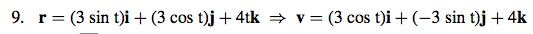# The derivative of ln cos x

## Homework Statement

the question is for plane curves. find T N and k for the plane curves

1. r(t) = ti + ln (cos t)

the derivative of ln x is 1/|x|, so why isn't the derivative of ln cos x, 1/cos x or sec x?

My book says the answer is - tan x

Last edited:

Because $\ln{\cos{x}}$ is actually a composition of the functions $\ln{x}$ and $\cos{x}$. Therefore you need to use the chain rule.

I put in the question into the original post. I don't see how they're using the chain rule. They aren't deriving two terms, it's just ln (cos t). if they're driving t, then i would think the derivative of t would just be 1.

you need to use the derivative of ln r(t), which is r'(t)/ r (t)
try that!

vela
Staff Emeritus
Homework Helper
Consider this problem: Find the derivative of ##y=\ln t^2##.

First method: Using the property of logs, we pull the exponent out front.
$$y=\ln t^2 = 2\ln t$$ When you differentiate this, you get
$$y' = 2\left(\frac{1}{t}\right).$$ This is the correct answer.

Second method: Just "differentiate" the way you did
$$y' = \frac{1}{t^2}.$$ This is wrong. Why is it wrong?

but there's no exponent involved here. plus look at this problem: they just derive everything normally here. they don't do the r'/rI like Serena
Homework Helper
The chain rule says that the derivative of f(g(t)) is f'(g(t))g'(t).
This is what vela illustrated.

In your case, for ln(cos(t)), you have calculated f'(g(t)) which is 1/cos(t), but you still need to multiply by g'(t) which is the derivative of cos(t).

ok, I sort of understand.

I like Serena
Homework Helper
ok, I sort of understand.

So do you understand how the book got -tan(t)?